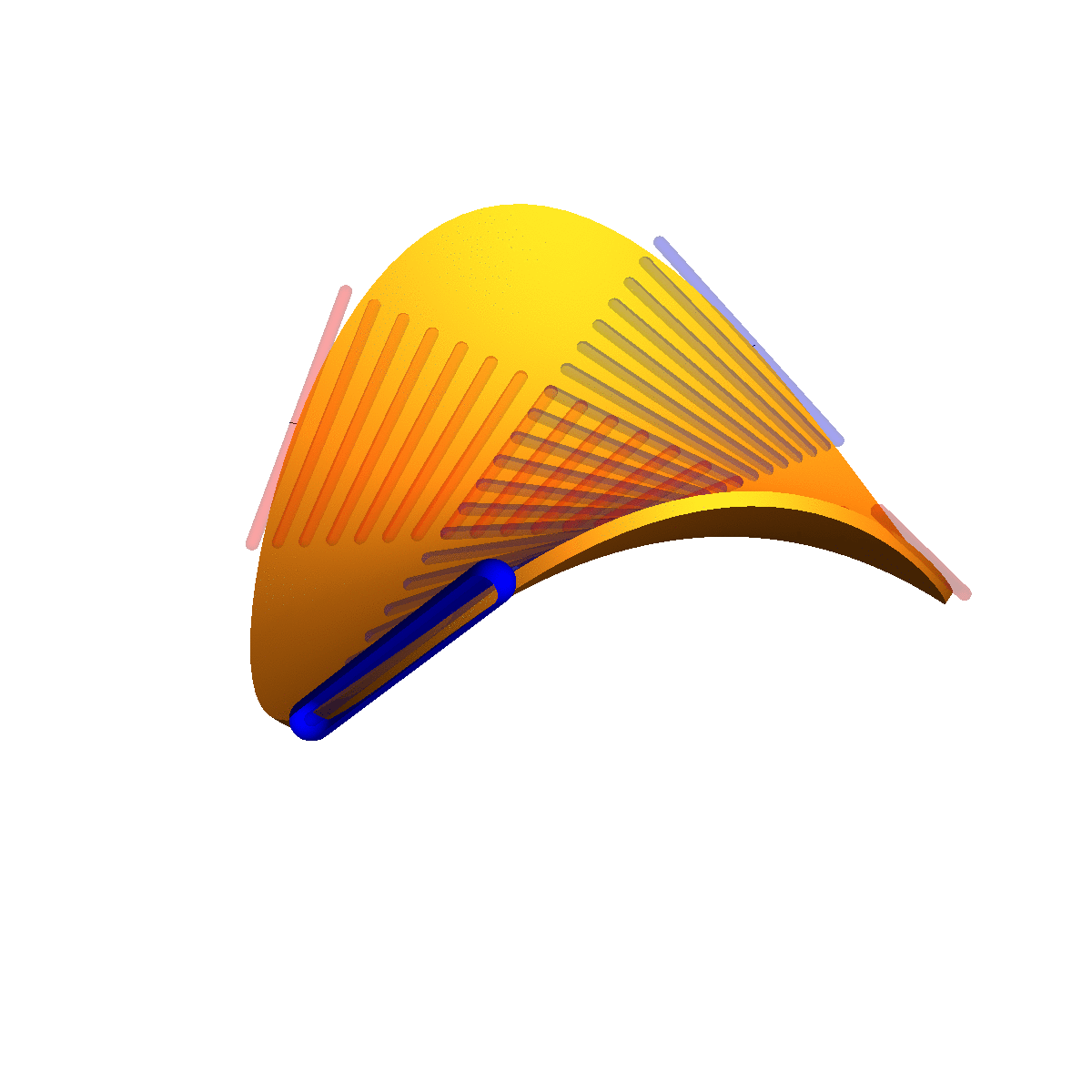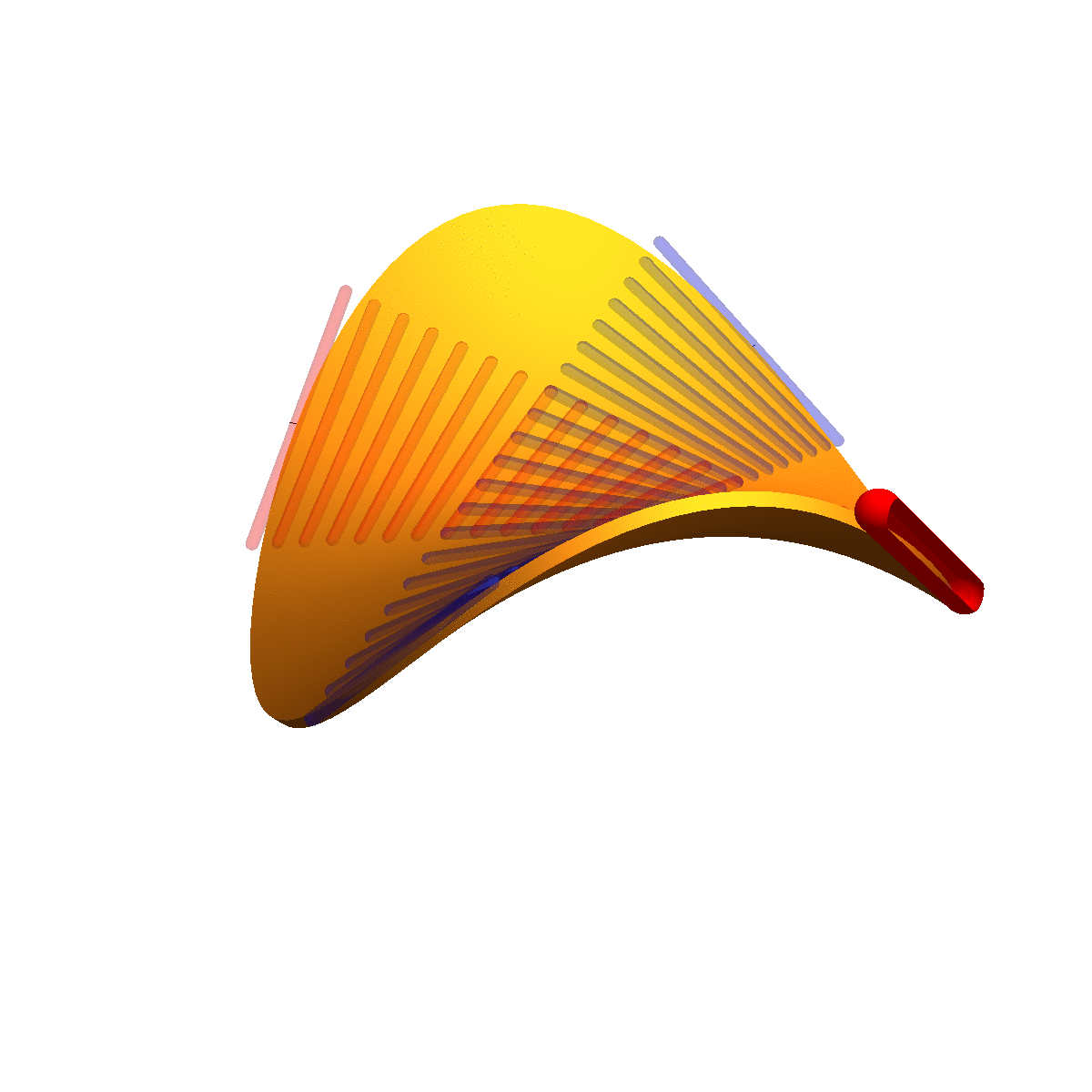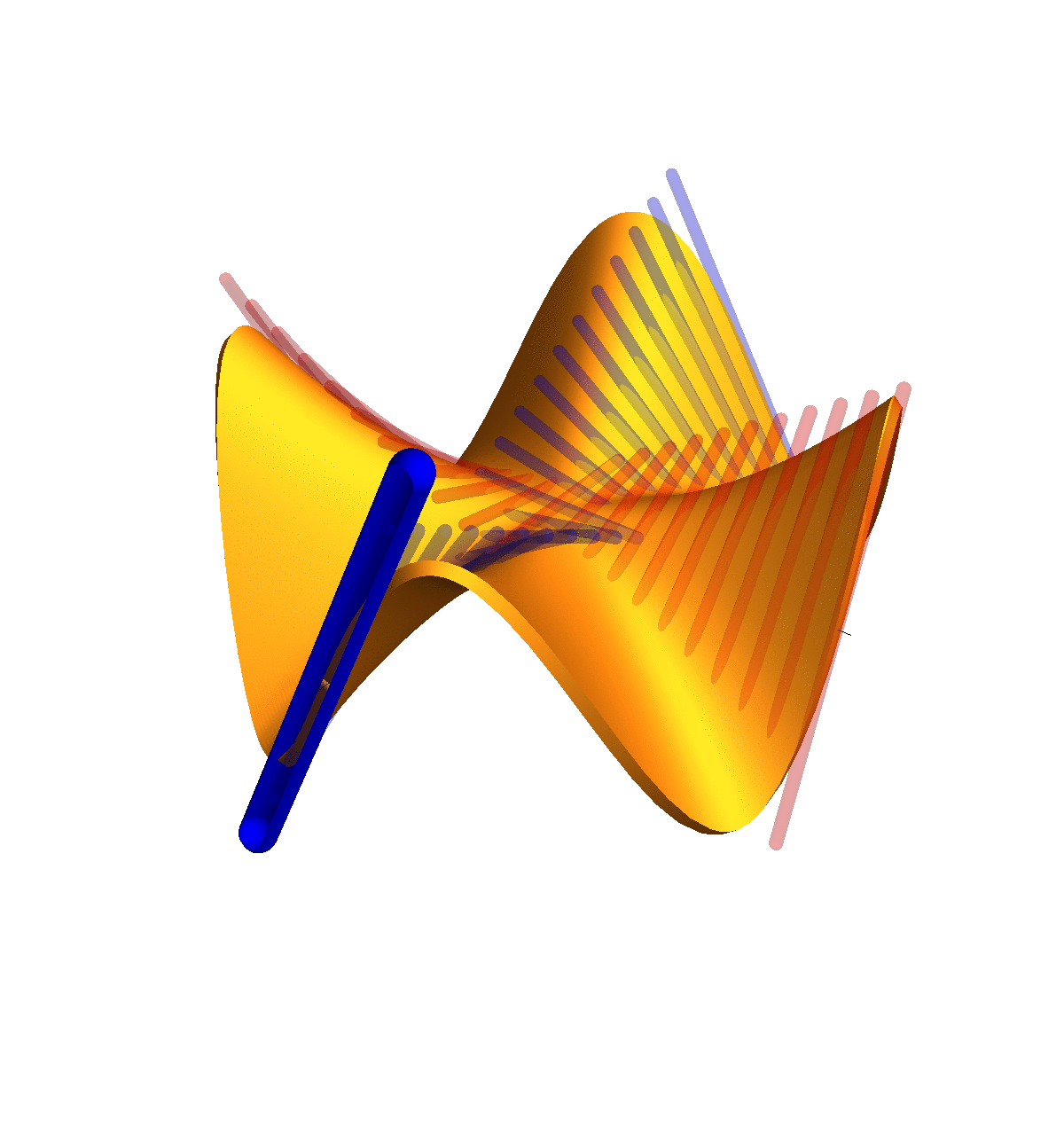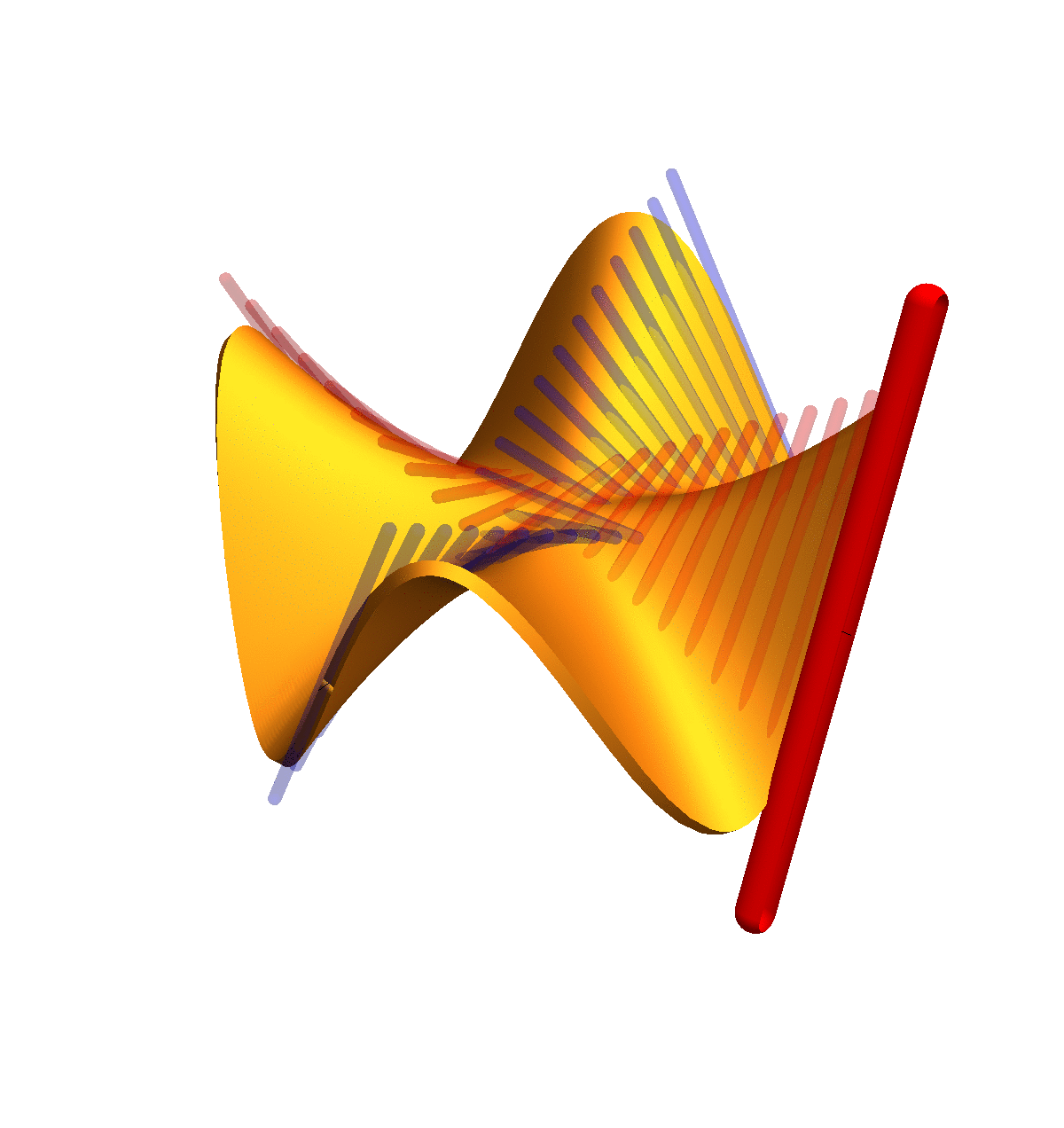# Clairaut counter example

We see here an illustration of Clairaut's theorem first for the function which is given in polar coordinates as
`g(r,t) = r2 sin(4t)`
and then for the function which is given in polar coordinates as
`f(r,t) = r2 sin(2t)`
We have proven in class that Clairaut's theorem holds. Thanks to Elliot who provided references to other proofs. There is a proof using the Stone-Weierstrass theorem Here (this is an approach I had considered as an alternative to the Fubini proof eventually prevailed as it allowed also to use the proof by contradiction and stating the fundamental theorem of calculus of variations assuring that if a continuous function has zero integrals over all cubes then it is zero. Stewart has a proof using the mean value theorem.)
 Clairaut theorem: f in C2 implies fxy=fyx. Clairaut counter example: there is g in C1 for which Clairaut fails
These two functions look very similar but only f is twice differentiable. The function g is only once differentiable. We can see that gxy and gyx are different. The function gx gives the slope in the x direction and gxy is the rate of change of that slope. The function gy gives the slope in the y direction and gyx gives the rate of change of that slope. You see that unlike for the function f, where the two rate of changes agree, in the second example for g, the rate of change has different sign.
 fxy fyxgxy gyx